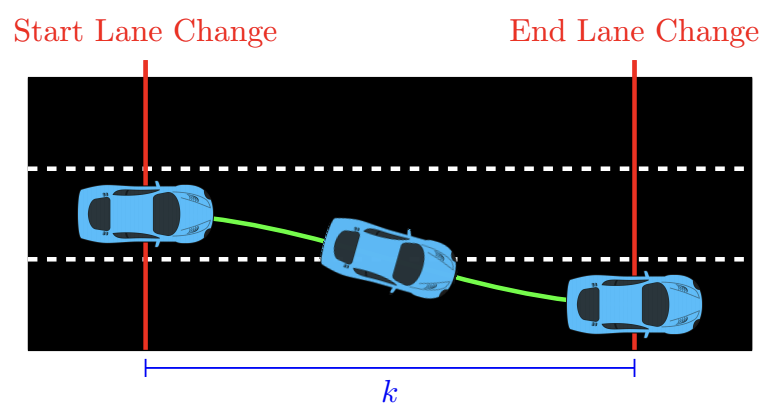시간 제한 메모리 제한 제출 정답 맞은 사람 정답 비율
2 초 512 MB 1 1 1 100.000%

## 문제

While driving around a curve on the highway, Sam realizes that if they use the inside lane, they travel a shorter distance. Sam wonders what is the minimum distance needed to travel to the destination.

The multilane highway consists of a sequence of straightaways that are connected by curves. When going around a curve, the distance travelled depends on which lane you are in. Each curve has a curvature $c$ and stretch $s$. Specifically, if Sam is in lane $i$, then they travel $s + c \cdot i$ meters while going around this curve.

Whenever Sam is on a straightaway, they may change from one lane into an adjacent lane.  When changing to an adjacent lane, Sam moves forward $k$ meters, but travels a total of $k+r$ meters.  Each lane change must be completed before the car reaches the end of the current straightaway.  Sam may change lanes multiple times in the same straightaway. For safety reasons, changing lanes is not possible on curves.Sam starts in lane $1$ and wishes to end in lane $1$. What is the minimum distance they must travel?

## 입력

The first line of input contains two integers $n$ ($1 \leq n \leq 250$), which is the number of straightaways, and $m$ ($1 \leq m \leq 250$), which is the number of lanes on the highway. The lanes are numbered $1, 2, \dots, m$.

The second line of input contains two integers $k$ ($1 \leq k \leq 10^6$) and $r$ ($1 \leq r \leq 10^6$), which are the lane changing parameters.

The next $n$ lines describe the straightaways in order. Each of these lines contains a single integer $\ell$ ($1 \leq \ell \leq 10^6$), which is the length of this straightaway.

The next $n-1$ lines describe the curves in order. Each of these lines contains two integers $s$ ($1 \leq s \leq 10^6$), which is the stretch of this curve, and $c$ ($-10^6 \leq c \leq 10^6$), which is the curvature of this curve. It is guaranteed that $s + c \cdot m > 0$.

The $i$th curve connects the $i$th and $(i+1)$th straightaway.

## 출력

Display the minimum distance Sam must travel.

## 예제 입력 1

4 3
5 2
10
10
10
10
4 -1
4 -1
4 1


## 예제 출력 1

51


## 예제 입력 2

4 3
5 2
10
10
10
10
10 -3
10 -3
10 1


## 예제 출력 2

61